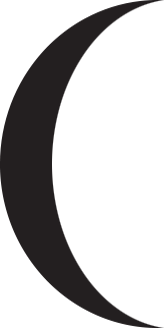# Help with cubicCurveTo to make desired shape

I need to use the class Graphics commands to make the shape shown below which is two curves with the same end points but different curvatures and the space between the curves filled in. The amount of curvature varies greatly so I need to draw the shape with code. As far as I can figure out, I need to use cubicCurveTo to draw the two curves and then endFill to fill the inside. The documentation talks about end points and control points but does not explain mathematically in any way how control points affect the curve. I’ve tried experimenting with different values and never get anything close to a curve. Can someone explain the math behind the control points or give me at least one example using endpoints (0,0) and (0,320) values and coords for two control points that actually produces a curve?